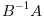Change language to:
English - Français - 日本語 - Русский

See the recommended documentation of this function

Ajuda do Scilab >> Dicas de Conversão de Matlab para Scilab > Matlab-Scilab equivalents > E > eig (Matlab function)

# eig (Matlab function)

Find eigenvalues and eigenvectors

### Matlab/Scilab equivalent

 Matlab Scilab `eig` `spec ; bdiag`

### Particular cases

eig(A)

Scilab equivalent for eig(A) is spec(A). Scilab eigenvector matrix can differ from Matlab one.

eig(A,'nobalance')

There is no Scilab equivalent for "nobalance" option. See examples.

eig(A,B,flag)

There is no Scilab equivalent for flag.If matrix `B` is nonsingular, the generalized eigenvalues can be computed as the eigenvalues of the matrixi.e. `d=spec(B^-1*A)`.If `A` is a real random square matrix, Scilab equivalent for the Matlab syntax `[V,D] = eig(A,'balance')` is `[D,V] = bdiag(A+%i*0, 1/%eps)`. In this case `D` consists of complex 1x1 blocks.
 Matlab Scilab ```d = eig(A,'balance') [V,D] = eig(A,'balance') [V,D] = eig(A,'balance') d = eig(A,B) d = eig(A,B) d = eig(A,B) [V,D] = eig(A,B)``` ```d = spec(A) [D,V]=bdiag(A) [V,D] = spec(A) [al,be] = spec(A,B); d = al./be; d = spec(A,B) d = spec(inv(B)*A) [al,be,V] = spec(A,B); D = diag(al./be);```